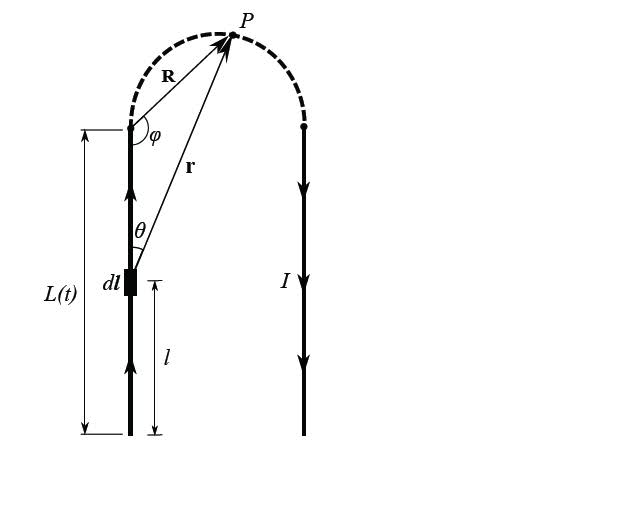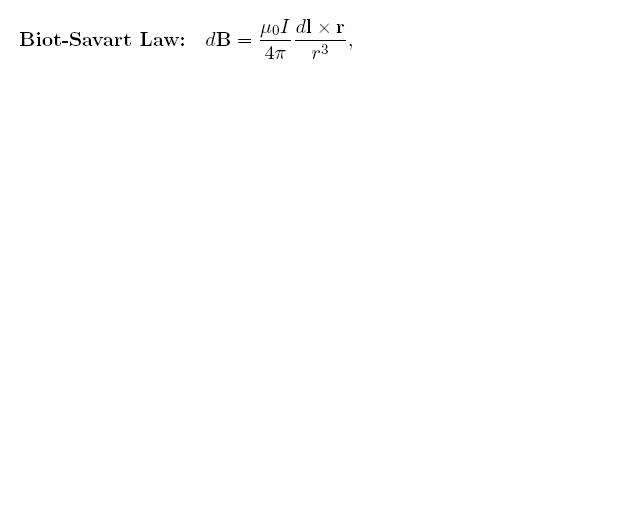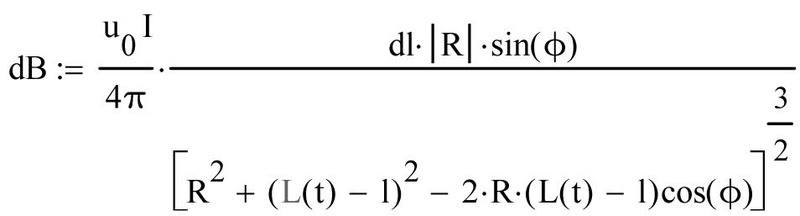# Expressing Biot-Savart Law using diagram

## Homework StatementExpress:in terms of R, L(t), l, and φ

## Homework Equations

Law of Sines and Cosines

## The Attempt at a Solution

I have used:
c^2=a^2+b^2-2ab*cosφ which resulted in
r^2=R^2+(L(t)-l)^2-2*R*(L(t)-l)*cosφ

and used the law of sines to get
R*sinφ=r*sinθ
r=(R*sinφ)/sinθ

I'm struggling figuring out where to go from here. I know I'm looking to replace the dl x r portion of the Biot-Savart law. If anyone could help prod me in the right direction I'd appreciate it. Thanks

Redbelly98
Staff Emeritus
Homework Helper
It appears that you are supposed to integrate the Biot-Savart law to get B at point P, thought it does not say this explicitly.

You'll need to express the magnitude of dl x r, using the usual rule for cross-product magnitudes.

Two things are not clear to me:

Is the current only in the straight portions of wire? It mysteriously "disappears and reappears" at the beginning and end of the semicircle section?

and,

The distance between the two straight wires is not given (or equivalently, the diameter of the semicircle). It seems that B should depend on that.

Sorry, should of mentioned that it is a rail-gun problem and the distance separating the rails is 10cm and each rail is 2 meters long. Thus, current will be running through the curved portion of the wire since it represents the armature. It's a multi-step problem though so I was thinking that this question was only asking for the general formula.

I believe I figured out my problem. I was going about things in the entirely wrong direction.
I came up with:I solved this by simply solving for r using the law of cosines and plugging it into the r3 portion of Biot's Law then to solve for dl x r I used the law of sines. And just made sure to get everything in terms of only R, L(t), l, and ϕ.
Anyone care to verify that this is correct?

Redbelly98
Staff Emeritus
Homework Helper
Looks good, but you don't really need the absolute-value sign on R do you?

I was using it to express magnitude but I don't think it's necessary. Also, I'm supposed to integrate this with respect to dl over the length of the rail to find the magnetic field at point P by the one rail. Not exactly sure what the first step into getting this one rolling would be. I know the length of the rail would be L(t) but not much more.

Redbelly98
Staff Emeritus